# Inductive voltage divider

Inductive voltage dividers are made out of two inductors. One of the inductors is connected from the input to the output and the other one is connected from the output to ground. You can also use other components like resistors and inductors. You can find more information about these here:
Voltage divider
Capacitive voltage divider

### Formulas

The output voltage can be calculated with the following formula: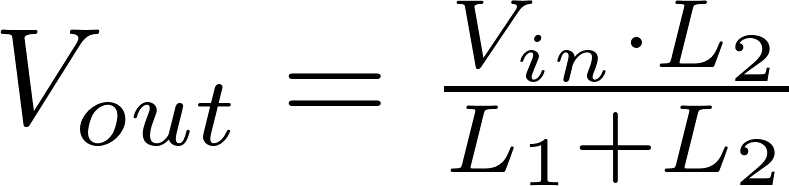The output voltage can be calculated with the following formula: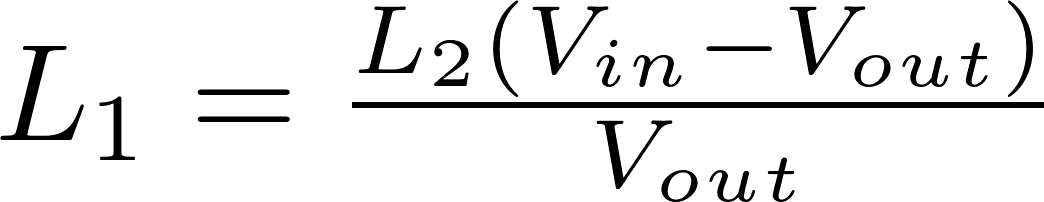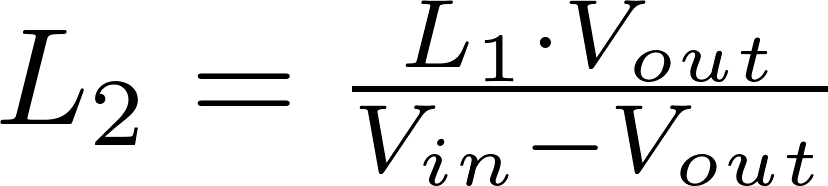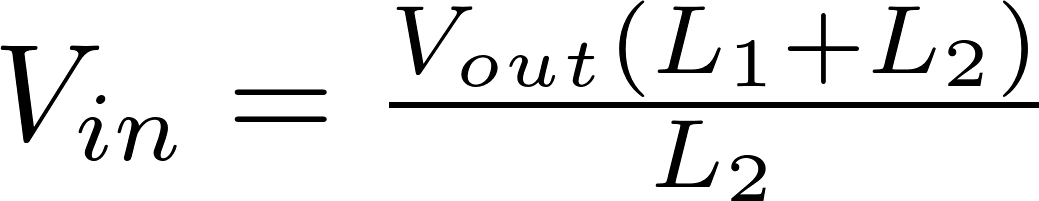L is the symbol for inductance and is measured in henry (H).
V in is the input voltage and is measured in volt (V)
V out is the output voltage and is measured in volt (V)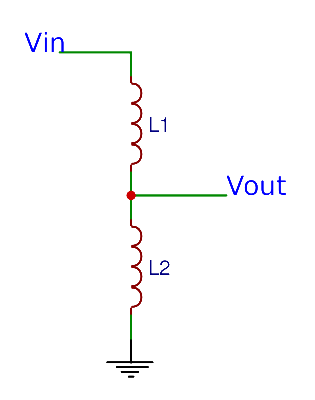### Calculator

Enter three values to calculate the remaining one.